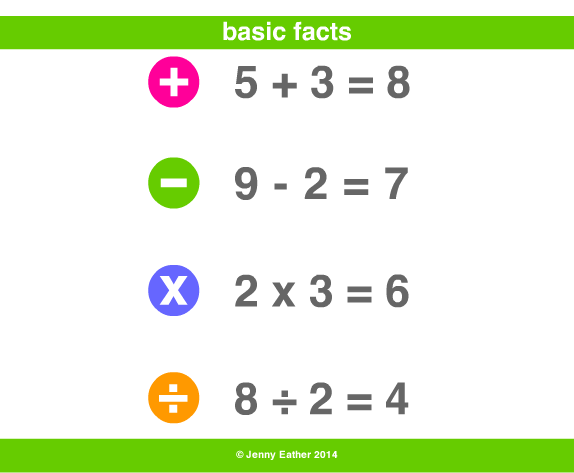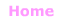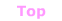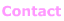Aa Bb Cc Dd Ee Ff Gg Hh Ii Jj Kk Ll Mm Nn Oo Pp Qq Rr Ss Tt Uu Vv Ww Xx Yy Zz

Bb

basic facts

• addition, subtraction, multiplication and division facts
mainly using one or two-digit numbers.

EXAMPLE:basic operations results

Subtraction (−)
minuend − subtrahend = difference

Multiplication (×)
multiplicand × multiplier = product

Division (÷)
dividend ÷ divisor = quotient© Jenny Eather 2014. All rights reserved.